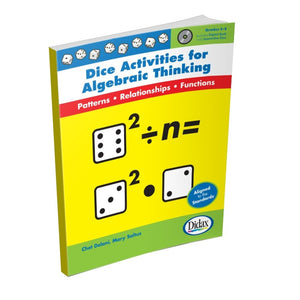# Didax Dice Activities for Algebraic Thinking Grades 5-8 (11396)

Regular price \$16.99 \$0.00

Didax Dice Activities for Algebraic Thinking Grades 5-8 (11396)

In this introduction to algebraic concepts, middle school students explore the idea of algebra as a "balancing act" using dice and colored tiles.
When given the opportunity to "play" with big mathematical ideas, students naturally acquire the algebra basics: using symbols to denote mathematical functions; using a variable (n) to represent an unknown; and writing and solving simultaneous equations.
These highly engaging team activities generate friendly competition, and provide a reason to learn and use algebraic concepts. These dice and tile activities address the CCSS of Number and Operations and Algebra as well as the Mathematical Practices of reasoning and problem solving.
136 pages.